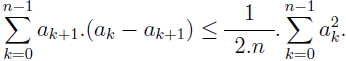# A modification of an elementary numerical inequality

Krassimir Atanassov
Notes on Number Theory and Discrete Mathematics, ISSN 1310-5132
Volume 18, 2012, Number 3, Pages 5—7

## Details

### Authors and affiliations

Krassimir AtanassovDept. of Bioinformatics and Mathematical Modelling
Institute of Biophysics and Biomedical Engineering, Bulgarian Academy of Sciences
105 Acad. G. Bonchev Str., 1113 Sofia, Bulgaria

### Abstract

It is proved that for every real numbers a0, a1, …, an (n ≥ 1):This is a modification of an inequality, previously introduced by the author.

### Keywords

• Arithmetic functions
• Inequalities

• 11A25

### References

1. Atanassov, K., An elementary numerical inequality. The Australian Mathematical Society Journal, Vol. 24, 1997, No. 5, 182
2. Beran, L., E. Novakova, On an inequality of Atanassov. The Australian Mathematical Society Journal, Vol. 25, 1998, No. 5, 234–235.
3. Coope, I., P. Renaud, A quadratic inequality of Atanassov. The Australian Mathematical Society Journal, Vol. 26, 1999, No. 4, 169–170.

## Cite this paper

APA

Atanassov, K. (2012). A modification of an elementary numerical inequality, Notes on Number Theory and Discrete Mathematics, 18(3), 5-7.

Chicago

Atanassov, Krassimir. “A modification of an elementary numerical inequality.” Notes on Number Theory and Discrete Mathematics 18, no. 3 (2012): 5-7.

MLA

Atanassov, Krassimir. “A modification of an elementary numerical inequality.” Notes on Number Theory and Discrete Mathematics 18.3 (2012): 5-7. Print.